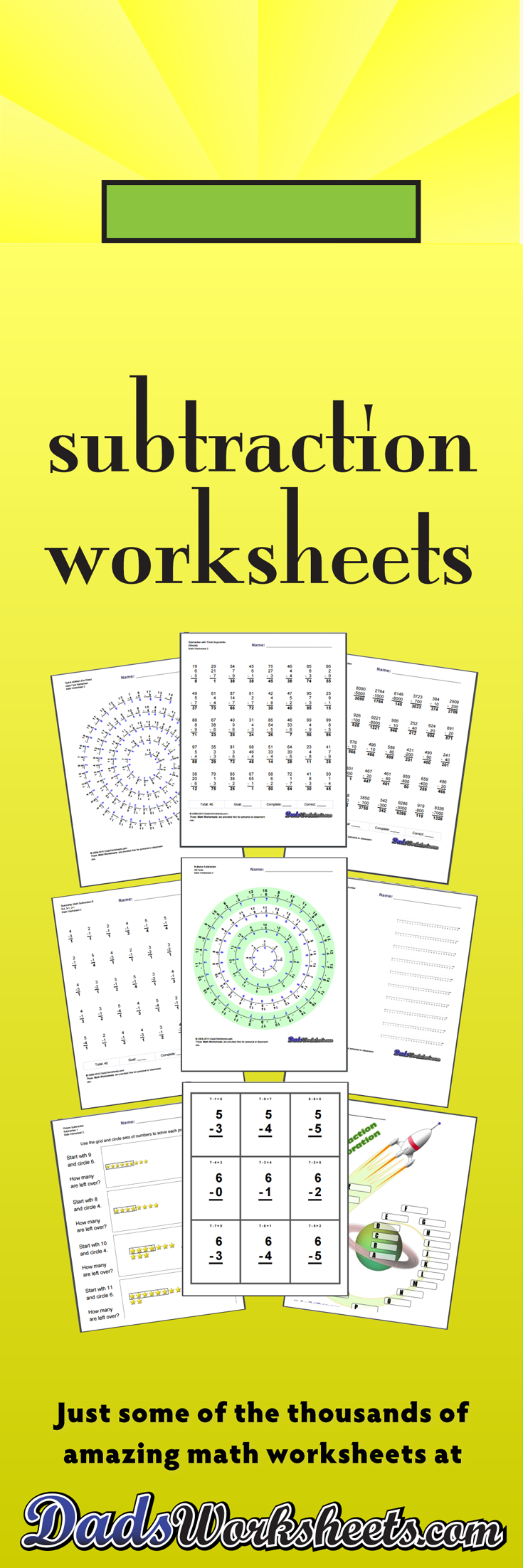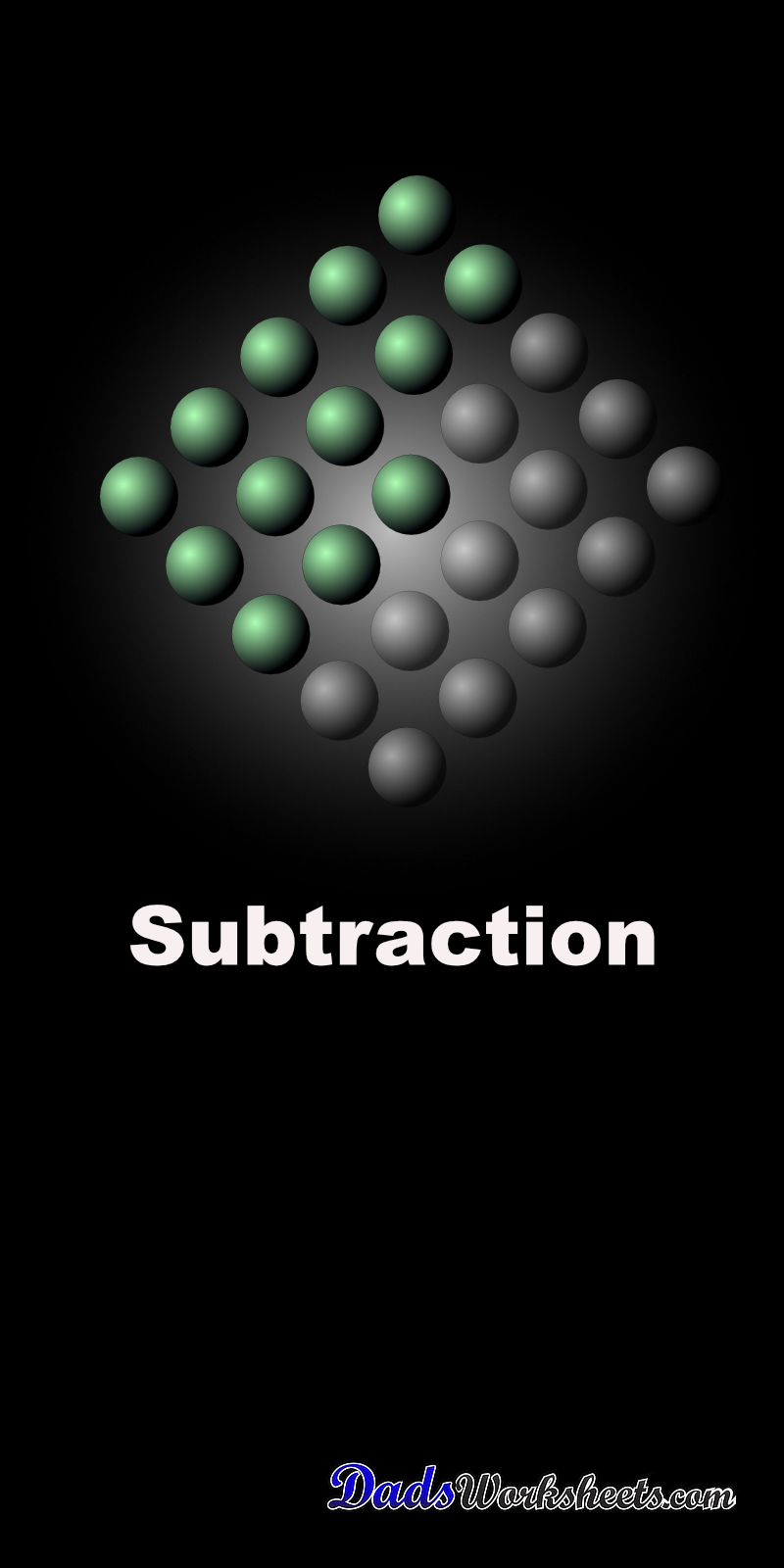One Dad. Four daughters. 9,251 worksheets... and counting!

# Subtraction Worksheets

These subtraction worksheets include timed math fact tests, multiple digit problems, subtraction with and without regrouping and much more. If you're looking for an incremental path from subtraction facts through basic multiple digit subtraction problems all the way to problems that are super-focused on subtraction regrouping, you've found everything you need! Try out the unique spiral and bullseye subtraction fact worksheets to get a completely different spin on your math fact practice. If I missed anything subtraction-related, please let me know!

## Subtraction with the Number Line

### 8 Subtraction Worksheets

These simple subtraction worksheets introduce subtraction concepts using the number Line.

## One Minute Timed Subtraction Worksheets

### 104 Subtraction Worksheets

The subtraction worksheets in this section are similar to the RocketMath, Mad Math Minutes or Mastering Math Facts subtraction worksheets used at many schools. These are typically one minute, timed subtraction tests. Try completing them with my super entertaining online timer if you or your kids are into silly sounds!

## Two Minute Tests

### 64 Subtraction Worksheets

These are two minute versions of the Spaceship Math subtraction worksheets with 80 and 100 problems per page.

## Circle Subtraction Facts Worksheets

### 24 Subtraction Worksheets

These Subtraction worksheets emphasize groups of related facts and there are variations with the facts in order to facilitate skip counting, or with random products that help facilitate fact memorization. Try the variations with all facts, or print the worksheets that focus only on specific families of multiplication facts that need more practice!

## Spiral Subtraction Facts

### 56 Subtraction Worksheets

Tired of the same old math fact worksheets with rows and rows of problems? Time to try something unique. These worksheets present the subtraction facts in a spiral layout that provides fun a twist on memorizing the basic math facts. They use the same fact layouts as the spaceship math sheets above, so try the first two sets worksheets if you are looking for the full set of subtraction facts or practice without the easier problems, or look at the others for an incremental approach to learning everything related to the trusty minus sign!

## Bullseye Subtraction

### 8 Subtraction Worksheets

Get on target with those subtraction math facts! These fun worksheets provide some additional kinesthetics over and above your usual math fact practice. These are great a great change to use as timed tests. Hopefully you will find these subtraction worksheets are spot-on when you need a change of pace!

## Place Value

### 8 Subtraction Worksheets

Place value subtraction worksheets. These primary worksheets are a good lead into the multiple digit subtraction worksheets.

## No Borrowing

### 24 Subtraction Worksheets

These multi-digit subtraction worksheets introduce problems without borrowing or regrouping.

## Borrowing Without Borrowing Across a Zero

### 24 Subtraction Worksheets

These worksheets are the next step in learning multiple digit subtraction. These multiple digit subtraction worksheets introduce borrowing (regrouping), but not borrowing across a zero.

## Borrowing Across Zero

### 24 Subtraction Worksheets

These subtraction worksheets focus on subtraction incorporating borrowing across zeroes. These are great subtraction worksheets to use to really practice borrowing if your student is struggling with regrouping concepts.

## Full Borrowing

### 44 Subtraction Worksheets

Multiple Digit Subtraction worksheets with a more conventional mix of problems (includes borrowing/regrouping.) See the worksheets in prior sections for more focused subtraction topics introducing multiple digits.

## Subtraction with Decimals

### 24 Subtraction Worksheets

Multi-digit subtraction worksheets that introduce decimals. This section includes sets of worksheets including subtraction with tenths, subtraction with hundredths and subtraction with thousandths.

## Three Argument Subtraction

### 16 Subtraction Worksheets

Subtraction worksheets with three arguments.

## Mixed Addition and Subtraction

### 16 Subtraction Worksheets

Math worksheets with mixed addition and subtraction problems. These problems help a lot to get students to pay attention to operations.

## Introduction to Negative Numbers

### 16 Subtraction Worksheets

Simple subtraction worksheets showing negative answers introduced with the number line. This is your starting point for negative numbers.

## Subtraction with Negative Results

### 28 Subtraction Worksheets

Practice worksheets with subtraction problems that may yield negative answers.

## Subtraction Worksheets for Math Practice!

Have you mastered the addition facts? Time to move on to subtraction! These subtraction fact timed tests and multiple digit problems introduce increasingly complex topics such as regrouping (borrowing/carrying) and you'll find plenty of multiple digit subtraction practice worksheets here to keep young minds engaged, but when you're ready for even more, be sure to check out these other math worksheets:
Picture Math Subtraction
Subtraction Flash Cards
Addition and Subtraction Grid Puzzle Worksheets